Fruits & Vegetables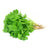Rs. 15.00
Rs. 60.00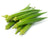Rs. 18.00
Rs. 25.00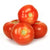Rs. 33.00
Rs. 60.00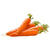Rs. 17.00
Rs. 30.00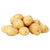Rs. 14.00
Rs. 22.00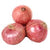Rs. 42.00
Rs. 49.00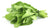Rs. 12.00
Rs. 45.00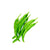Rs. 18.00
Rs. 20.00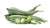Rs. 30.00
Rs. 49.00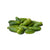Rs. 15.00
Rs. 24.00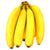Rs. 16.00
Rs. 20.00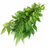Rs. 15.00
Rs. 20.00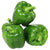Rs. 22.00
Rs. 35.00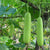Rs. 30.00
Rs. 37.50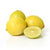Rs. 15.00
Rs. 26.00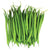Rs. 14.00
Rs. 35.00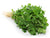Rs. 17.00
Rs. 25.00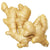Rs. 15.00
Rs. 35.00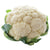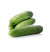Rs. 15.00
Rs. 25.00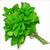Rs. 15.00
Rs. 22.00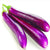Rs. 14.00
Rs. 23.50
Sold Out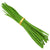Rs. 23.00
Rs. 30.00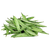Rs. 17.50
Rs. 30.00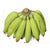Rs. 12.00
Rs. 19.00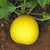Rs. 39.00
Rs. 45.00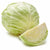Rs. 32.00
Rs. 52.50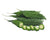Rs. 35.00
Rs. 42.00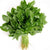Rs. 10.00
Rs. 35.00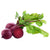Rs. 26.00
Rs. 32.00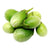Rs. 14.00
Rs. 23.50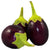Rs. 14.00
Rs. 22.50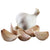Rs. 40.00
Rs. 65.00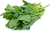Rs. 15.00
Rs. 45.00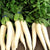Rs. 16.50
Rs. 22.00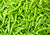Rs. 18.00
Rs. 20.00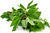Rs. 20.00
Rs. 28.00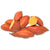Rs. 23.50
Rs. 28.00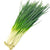Rs. 13.00
Rs. 16.25
Sold Out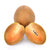Rs. 45.00
Rs. 60.00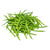Rs. 15.00
Rs. 29.50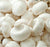Rs. 62.00
Rs. 69.00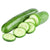Rs. 39.00
Rs. 46.50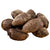Rs. 12.50
Rs. 19.00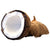Rs. 35.50
Rs. 42.00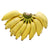Rs. 35.00
Rs. 58.00
Sold Out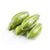Rs. 27.00
Rs. 45.00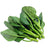Rs. 15.00
Rs. 45.00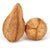Rs. 29.50
Rs. 35.00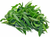Rs. 20.00
Rs. 24.00
• 1